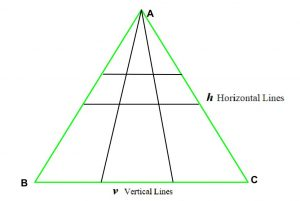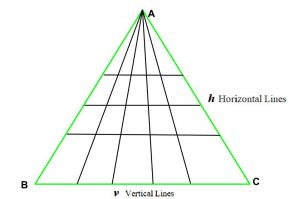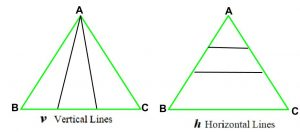Open in App
Not now

# Total number of triangles formed when there are H horizontal and V vertical lines

• Last Updated : 07 Apr, 2021

Given a triangle ABC. H horizontal lines from side AB to AC (as shown in fig.) and V vertical lines from vertex A to side BC are drawn, the task is to find the total no. of triangles formed.
Examples:

Input: H = 2, V = 2
Output: 18As we see in the image above, total triangles formed are 18.
Input: H = 3, V = 4
Output: 60Approach: As we see in the images below, we can derive a general formula for above problem:

1. If there are only h horizontal lines then total triangles are (h + 1).
2. If there are only v vertical lines then total triangles are (v + 1) * (v + 2) / 2.1. So, total triangles are Triangles formed by horizontal lines * Triangles formed by vertical lines i.e. (h + 1) * (( v + 1) * (v + 2) / 2).

Below is the implementation of the above approach:

## C++

 `// C++ implementation of the approach` `#include ` `using` `namespace` `std;` `#define LLI long long int`   `// Function to return total triangles` `LLI totalTriangles(LLI h, LLI v)` `{` `    ``// Only possible triangle is` `    ``// the given triangle` `    ``if` `(h == 0 && v == 0)` `        ``return` `1;`   `    ``// If only vertical lines are present` `    ``if` `(h == 0)` `        ``return` `((v + 1) * (v + 2) / 2);`   `    ``// If only horizontal lines are present` `    ``if` `(v == 0)` `        ``return` `(h + 1);`   `    ``// Return total triangles` `    ``LLI Total = (h + 1) * ((v + 1) * (v + 2) / 2);`   `    ``return` `Total;` `}`   `// Driver code` `int` `main()` `{` `    ``int` `h = 2, v = 2;` `    ``cout << totalTriangles(h, v);`   `    ``return` `0;` `}`

## Java

 `// Java implementation of the approach` `class` `GFG {`   `    ``// Function to return total triangles` `    ``public` `static` `int` `totalTriangles(``int` `h, ``int` `v)` `    ``{` `        ``// Only possible triangle is` `        ``// the given triangle` `        ``if` `(h == ``0` `&& v == ``0``)` `            ``return` `1``;`   `        ``// If only vertical lines are present` `        ``if` `(h == ``0``)` `            ``return` `((v + ``1``) * (v + ``2``) / ``2``);`   `        ``// If only horizontal lines are present` `        ``if` `(v == ``0``)` `            ``return` `(h + ``1``);`   `        ``// Return total triangles` `        ``int` `total = (h + ``1``) * ((v + ``1``) * (v + ``2``) / ``2``);`   `        ``return` `total;` `    ``}`   `    ``// Driver code` `    ``public` `static` `void` `main(String[] args)` `    ``{` `        ``int` `h = ``2``, v = ``2``;` `        ``System.out.print(totalTriangles(h, v));` `    ``}` `}`

## C#

 `// C# implementation of the approach ` `using` `System;`   `class` `GFG ` `{ `   `    ``// Function to return total triangles ` `    ``public` `static` `int` `totalTriangles(``int` `h, ``int` `v) ` `    ``{ ` `        ``// Only possible triangle is ` `        ``// the given triangle ` `        ``if` `(h == 0 && v == 0) ` `            ``return` `1; `   `        ``// If only vertical lines are present ` `        ``if` `(h == 0) ` `            ``return` `((v + 1) * (v + 2) / 2); `   `        ``// If only horizontal lines are present ` `        ``if` `(v == 0) ` `            ``return` `(h + 1); `   `        ``// Return total triangles ` `        ``int` `total = (h + 1) * ((v + 1) * (v + 2) / 2); `   `        ``return` `total; ` `    ``} `   `    ``// Driver code ` `    ``public` `static` `void` `Main() ` `    ``{ ` `        ``int` `h = 2, v = 2; ` `        ``Console.Write(totalTriangles(h, v)); ` `    ``} ` `} `   `// This code is contributed by Ryuga`

## Python3

 `# Python3 implementation of the approach`   `# Function to return total triangles` `def` `totalTriangles(h, v):` `    `  `    ``# Only possible triangle is ` `    ``# the given triangle` `    ``if` `(h ``=``=` `0` `and` `v ``=``=` `0``):` `        ``return` `1`   `    ``# If only vertical lines are present` `    ``if` `(h ``=``=` `0``):` `        ``return` `((v ``+` `1``) ``*` `(v ``+` `2``) ``/` `2``)`   `    ``# If only horizontal lines are present` `    ``if` `(v ``=``=` `0``):` `        ``return` `(h ``+` `1``)`   `    ``# Return total triangles` `    ``total ``=` `(h ``+` `1``) ``*` `((v ``+` `1``) ``*` `(v ``+` `2``) ``/` `2``)`   `    ``return` `total`   `# Driver code` `h ``=` `2` `v ``=` `2` `print``(``int``(totalTriangles(h, v)))`

## PHP

 ``

## Javascript

 ``

Output:

`18`

Time Complexity: O(1)
Auxiliary Space: O(1)

My Personal Notes arrow_drop_up
Related Articles